# Fixed-point property is retract-hereditary

This article gives the statement, and possibly proof, of a topological space property (i.e., fixed-point property) satisfying a topological space metaproperty (i.e., retract-hereditary property of topological spaces)
View all topological space metaproperty satisfactions | View all topological space metaproperty dissatisfactions
Get more facts about fixed-point property |Get facts that use property satisfaction of fixed-point property | Get facts that use property satisfaction of fixed-point property|Get more facts about retract-hereditary property of topological spaces

## Statement

### Verbal statement

Any retract of a topological space having the fixed-point property, also has the fixed-point property.

## Proof

### Proof outline

• Consider a self-map of the retract
• Compose with the retraction to get a self-map of the whole space
• Find a fixed point, and observe that it must be a fixed point of the original self-map

### Proof details

Given: A topological space$X$ satisfying the fixed-point property, a retraction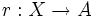$r:X \to A$ where$A \subset X$ and$r(a) = a$ for all$a \in A$

To prove:$A$ satisfies the fixed-point property

Proof: Let$i$ denote the inclusion of$A$ in$X$.

Consider any continuous map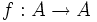$f:A \to A$. We need to show that$f$ has a fixed point in$A$. Consider the composition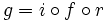$g = i \circ f \circ r$. This is a map from$X$ to$X$ that first retracts to$A$, then applies$f$, and then views the resulting point of$A$ as a point in$X$.$g$ is a composite of continuous maps, so$g$ is continuous. Since$X$ has the fixed-point property, there exists$x \in X$ such that$g(x) = x$.

But by construction,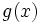$g(x)$ is actually inside$A$, so in fact$x \in A$. But if$x \in A$,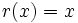$r(x) = x$, so we conclude that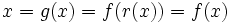$x = g(x) = f(r(x)) = f(x)$. Thus,$x \in A$ is a fixed point of$f$, completing the proof.